Theory.org » software » qfe » Quaternion Fractal Explorer (QFE)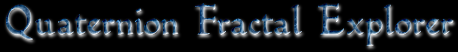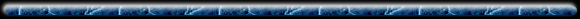This was origionally something I cooked up as a project for my graphics class in fall 97. I've been working on it off and on ever since.

The idea is to create an interactive quaternion fractal viewer, a 3d fractint if you will. Quaternions are a generalization of traditional complex numbers; every complex number is a degenerate quaternion. I've implemented Jules Bloomenthal's implicit polygonization algorithm. You can see the results below. Each point is colored by taking the absolute value of the individual components of the normal and using these for the red, blue and green values.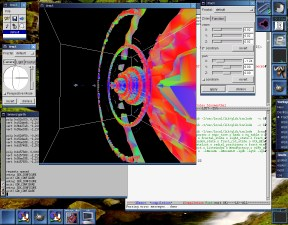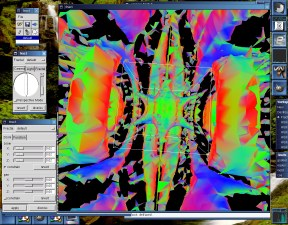Here is the quartic mandelbrot set (f(q)=q4+c). The camera is pointing at a secondary mandelbrot set in the nose. The large rings are other secondary mandelbrot sets which do not lie on the real axis. It seems that quaternion mandelbrot sets exhibit radial symmetry around the real axis. This is a cubic julia set,(f(q)=q3+[0.22374 -1.21619 0 0]). This is the same function used to generate the complex julia set illustrated here. The view is from within the main 'bulb' of the set. The images below are more recent screenshots. The coloring method is different - the images above color each point by the abolute value of the normal components. Images below use (normal+1)/2 instead. This makes opposing vectors different colors, and seems to show what's going on a little better. I've also included an XaoS window showing the complex counterpart in each case.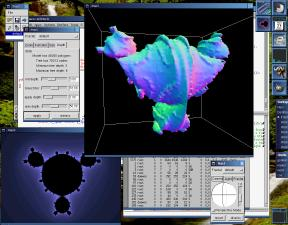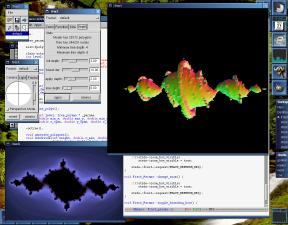This is another cubic julia set, this time generated with the function f(q)=q3+[0 -0.916666666 0 0]. The constant is from the primary mandelbrot set, hence this set is less hectic than the one above. To the lower left is the complex representation of the same set, courtesy of XaoS. This is a quadratic julia set, f(q)=q2+[-1 -0.13 0 0].

The interface has been done with gtk-- and openGL. The shots above were taken under Linux with mesa. If you would like to try it for yourself, beware that the code is not in the best state right now. If that hasn't deterred you, go ahead and grab a copy of the latest cvs snapshot. You will also need a copy of libquat. If you would like (possibly) more recent copies you can try the theory.org anon cvs.If you find this sort of thing interesting you should check out the following links:

Skal's 3D-fractals collection
Pascal Massimino's page. Much about quaternion fractals and raytracing. Mmm...

Quat 0.91
This is a cute little quaternion ray tracer. Very nicely done.

Stewart Dickson: Portfolio of 3D Fractal Works
Some interesting quaternion fractal stuff here.

How-to: Quaternions
A good reference

Quaternion Julia Set VRML Server
This site was my inspiration for using Jules Bloomenthal's implicit surface polygonization algorithm. It renders quadratic quaternion julia sets to vrml with it. Pretty cool.Brian Martin :: brian@theory.org